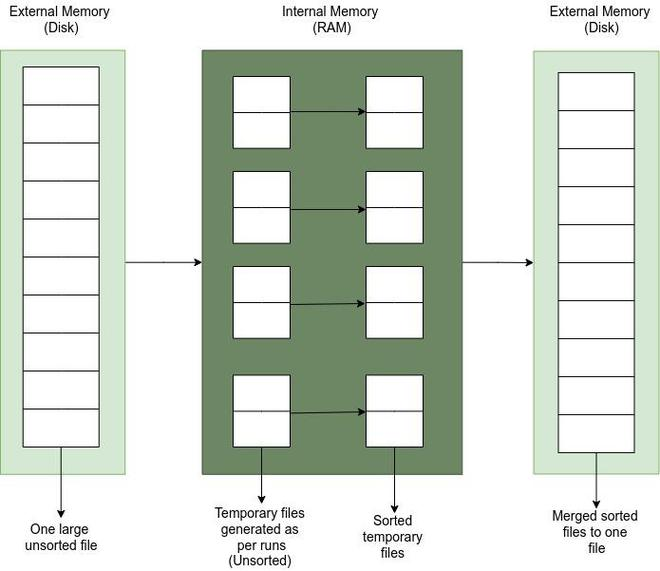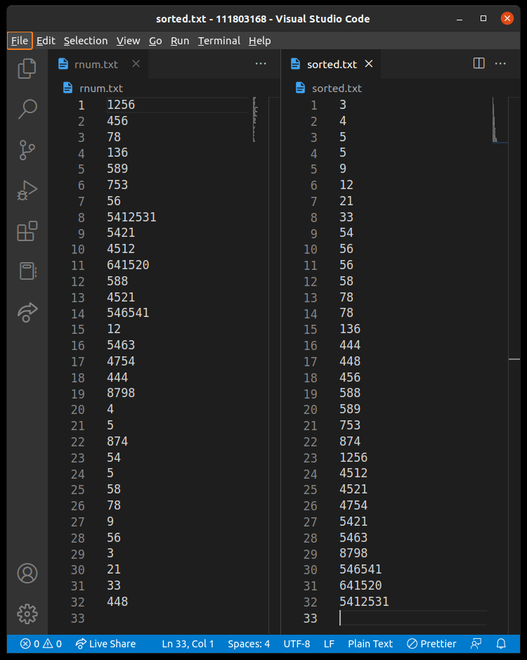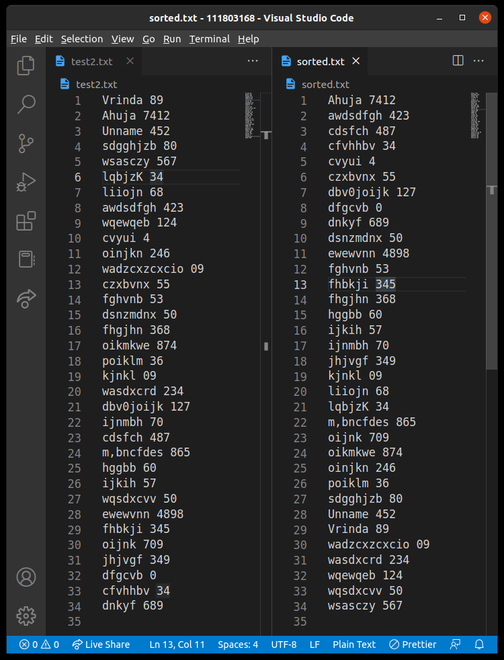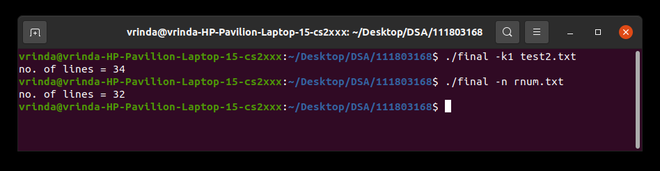Open in App
Not now

# Internal Implementation of Linux Sort Command

• Last Updated : 27 Nov, 2022

Prerequisite:  SORT command in Linux/Unix with examples

The SORT command in the Linux operating system is used for sorting. It can be done either for files or for input on a command line given by the user. The sort command by default sorts files under the assumption that their data is ASCII. It sorts the files line by line.

The SORT command follows a variety of features for the output. The first is that the alphabetical lines will follow the lines with numbers. Lines containing lowercase letters will appear before lines containing the same character in uppercase. The SORT command has several options for sorting in various ways. Some of them include:

• -k: This option sorts the file based on the key number, which is stated in the option after k. For example, the “-k2” option would sort the file using the second column of the file.
• -n: This option sorts the file numerically since the default behavior is ASCII based.
• -b: This option ignores the leading blanks in the file while sorting.
• -d: This option sorts according to the dictionary order.
• -r: This option does a reverse sort or reverses the sorted result.
• -m: This option merges the already sorted files given as input.
• -u: This option removes duplicates in the file and then sorts it.

The SORT command can be used to sort large files that cannot fit in the main memory and thus reside in the external memory using the mechanism of external sorting, which is a class of sorting algorithms that can handle enormous volumes of data. The algorithm can be more specifically termed External Merge Sorting, and it essentially operates by first merging the sorted chunks together after it sorts the chunks into groups that can all fit in RAM.

Simply put, first divide the file into runs that fit in the main memory, then sort each run in the main memory, and finally merge the sorted runs into the external memory.External Sorting

## Steps for Internal Implementation of Linux Sort Command

The implementation of the SORT command differs a little on the basis of the option mentioned, but the primary steps remain the same. The implementation uses the external merge sorting algorithm as mentioned above. The steps for the implementation are as follows:

1. First, we calculate the number of runs for sorting. This is calculated by dividing the number of lines in the file by the size of the main memory.
2. Based on the number of runs and size of the main memory we then perform external sorting by two broad steps:
• Creating temporary files and sorting them
• Merging sorted files

Explaining the second and most important step in detail:

1. For creating temporary files from the main file, the number of runs, the size of the main memory, and the number of lines in the file are required. Initially, we malloc an array of File pointers and character arrays for filenames according to the number of runs needed. The last run is to be treated differently since it can also contain a lesser number of characters than the other files. Now for each temp file pointer in the array, a file is malloced and opened with “w+” mode. In the same loop, data is written into the temporary file from the main file, character by character. The remaining data is written in the last temporary file.
2.  After creating input temporary files, in a similar way output temporary files are created to store the sorted temporary file data. In each loop, an output temporary file is created for the corresponding input temporary file and it is then sorted by performing three operations:
1. Readline: Reads the input temporary file line by line and stores the data in the malloced pointer of the structure line
2. Column Separate: This is basically used due for sorting by option “-k” to sort the file based on key number i.e., a specific column number. The default value of the key number is set to 1, so it means sorting takes place based on the first column of the file. In this function, the data is separated column-wise and stored in another 2D array according to the key number
3. MergeSort: Here the actual sorting of the arrays takes place and the temporary files are thus sorted
3. After sorting the temporary files individually, they need to be merged in a sorted manner and then get stored in a single file. This is done by merge sort using a heap. Implementation of heap i.e., methods like heapInit, heapInsert, heapRemove is also done according to the structures used and required.

The primary steps for implementing the sort command remain almost the same. There are some specific changes depending on the options given to the sort command. Some of them include the following:

• For the “-k” option, a particular key number is provided based on which the sorting is done
• For the “-r” option, sorting needs to be done in a reverse manner. This can either be done by actually sorting in reverse or sorting normally and in the end reversing the output.
• For the “-m” option, the files are already sorted so we only need to call the merging sorted files method.
• For the “-n” option, sorting is performed according to numeric order and not ASCII

## Code Snippets for Implementation in the C Language

Creating temporary files and filenames for them:

## C

 `temp = (``FILE``**)``malloc``(``sizeof``(``FILE``*) * runs); ` `filename = (``char``**)``malloc``(``sizeof``(``char``*) * runs); ` `for` `(i = 0; i < runs; i++) { ` `    ``filename[i] = (``char``*)``malloc``(``sizeof``(``char``) * 3); ` `} ` `i = 0; ` `while` `(i < runs - 1) { ` `    ``temp[i] = (``FILE``*)``malloc``(``sizeof``(``FILE``)); ` `    ``temp[i] = openFile(filename1[i], ``"w+"``); ` `    ``writefile(mainFile, temp[i], main_mem); ` `    ``fclose``(temp[i]); ` `    ``i++; ` `} ` `temp[i] = (``FILE``*)``malloc``(``sizeof``(``FILE``)); ` `temp[i] = openFile(filename1[i], ``"w+"``); ` `writefile(mainFile, temp[i], lastrun); ` `fclose``(temp[i]);`

Writing data to the temporary file from the main file character by character:

## C

 `void` `writefile(``FILE``* mainFile, ``FILE``* tempFile, ``int` `numLines) ` `{ ` `    ``char` `c; ` `    ``int` `i; ` `    ``for` `(i = 0; i < nline; i++) { ` `        ``fscanf``(mainFile, ``"%c"``, &c); ` `        ``while` `(c != ``'\n'``) { ` `            ``fprintf``(tempFile, ``"%c"``, c); ` `            ``fscanf``(mainFile, ``"%c"``, &c); ` `        ``} ` `        ``fprintf``(tempFile, ``"%c"``, c); ` `    ``} ` `}`

Creating output temporary files and sorting the individual temporary files:

## C

 `for` `(i = 0; i < runs - 1; i++) { ` `    ``out[i] = (``FILE``*)``malloc``(``sizeof``(``FILE``)); ` `    ``out[i] = openFile(filename2[i], ``"w+"``); ` `    ``temp[i] = openFile(filename1[i], ``"r+"``); ` `    ``readLine(temp[i]); ` `    ``columnSeparate(main_mem, op); ` `    ``mergeSort(op, 0, main_mem - 1); ` `    ``free``(l); ` `    ``free``(b); ` `    ``free``(d); ` `    ``fclose``(temp[i]); ` `    ``fclose``(out[i]); ` `}`

## C

 `void` `readLine(``FILE``* fp) ` `{ ` `    ``int` `j = 0, i, f, g, n; ` `    ``char` `c; ` `    ``n = main_mem; ` `    ``l = (line*)``malloc``(``sizeof``(line) * n); ` `    ``b = (``char``**)``malloc``(``sizeof``(``char``*) * (n)); ` `    ``for` `(i = 0; i < n; i++) { ` `        ``b[i] = (``char``*)``malloc``(``sizeof``(``char``) * 256); ` `    ``} ` `    ``d = (``char``**)``malloc``(``sizeof``(``char``*) * (n)); ` `    ``for` `(i = 0; i < n; i++) { ` `        ``d[i] = (``char``*)``malloc``(``sizeof``(``char``) * 256); ` `    ``} ` `    ``for` `(i = 0; i < n; i++) { ` `        ``l[i].temp = (``char``*)``malloc``(``sizeof``(``char``) * 256); ` `        ``g = ``fscanf``(fp, ``"%c"``, &l[i].temp[j]); ` `        ``while` `(l[i].temp[j] != ``'\n'``) { ` `            ``j++; ` `            ``f = ``fscanf``(fp, ``"%c"``, &(l[i].temp[j])); ` `        ``} ` `        ``l[i].temp[j] = ``'\0'``; ` `        ``j = 0; ` `    ``} ` `}`

Column Separate function:

## C

 `void` `columnSeparate(``int` `lines, opt op) ` `{ ` `    ``char` `tem, str; ` `    ``int` `i = 0, j 0, count, k = 0, len; ` `    ``int` `col; ` `    ``if` `(op.keyno == 1) { ` `        ``for` `(i = 0; i < lines; i++) { ` `            ``len = ``strlen``(l[i].temp); ` `            ``while` `(j < len) { ` `                ``tem[k] = l[i].temp[j]; ` `                ``k++; ` `                ``j++; ` `                ``if` `(l[i].temp[j] == ``'\0'``) { ` `                    ``break``; ` `                ``} ` `            ``} ` `            ``tem[k] = ``'\0'``; ` `            ``j = 0, k = 0; ` `            ``l[i].col = (``char``*)``malloc``(``sizeof``(``char``) * 256); ` `            ``strcpy``(l[i].col, tem); ` `            ``strcpy``(tem, ``""``); ` `        ``} ` `    ``} ` `    ``else` `{ ` `        ``for` `(i = 0; i < lines; i++) { ` `            ``len = ``strlen``(l[i].temp); ` `            ``while` `(j < (len - 1)) { ` `                ``if` `(l[i].temp[j] == ``' '` `                    ``&& l[i].temp[j + 1] != ``' '` `                    ``&& count == 0) { ` `                    ``col++; ` `                    ``if` `(col == op.keyno) { ` `                        ``count = 1; ` `                        ``break``; ` `                    ``} ` `                ``} ` `                ``else` `                    ``j++; ` `            ``} ` `            ``j++; ` `            ``while` `(l[i].temp[j] != ``'\0'` `                   ``&& l[i].temp[j] != ``' '` `&& count == 1) { ` `                ``tem[k] = l[i].temp[j]; ` `                ``k++; ` `                ``j++; ` `            ``} ` `            ``if` `(count == 1) { ` `                ``tem[k] = ``'\0'``; ` `                ``j = k = col = count = 0; ` `                ``l[i].col ` `                    ``= (``char``*)``malloc``(``sizeof``(``char``) * 256); ` `                ``strcpy``(l[i].col, str); ` `                ``strcpy``(str, ``""``); ` `            ``} ` `        ``} ` `    ``} ` `}`

Merging the sorted files using Heap functions and storing them in one output file:

## C

 `void` `mergeSortedFiles(``int` `n, ``int` `k, opt op) ` `{ ` `    ``FILE` `**out, *fp; ` `    ``char` `**filename, str; ` `    ``out = (``FILE``**)``malloc``(``sizeof``(``FILE``*) * k); ` `    ``filename = (``char``**)``malloc``(``sizeof``(``char``*) * k); ` `    ``data d; ` `    ``int` `no, temp[k], i, j = 0, count = 0; ` `    ``heapinit(&h); ` `    ``fp = openFile(``"sorted.txt"``, ``"w+"``); ` `    ``for` `(i = 0; i < k; i++) { ` `        ``filename[i] = (``char``*)``malloc``(``sizeof``(``char``) * 5); ` `        ``sprintf``(filename[i], ``"%d"``, i + 500); ` `    ``} ` `    ``for` `(i = 0; i < k; i++) { ` `        ``out[i] = (``FILE``*)``malloc``(``sizeof``(``FILE``)); ` `        ``out[i] = openFile(filename[i], ``"r+"``); ` `    ``} ` `    ``for` `(i = 0; i < k; i++) { ` `        ``l = (line*)``malloc``(``sizeof``(line)); ` `        ``l.temp = (``char``*)``malloc``(``sizeof``(``char``) * 256); ` `        ``j = 0; ` `        ``fscanf``(out[i], ``"%c"``, &l.temp[j]); ` `        ``while` `(l.temp[j] != ``'\n'``) { ` `            ``j++; ` `            ``fscanf``(out[i], ``"%c"``, &l.temp[j]); ` `        ``} ` `        ``l.temp[j] = ``'\0'``; ` `        ``columnseperate(1, op); ` `        ``heapinsert(&h, l.col, i, op, l.temp); ` `        ``free``(l); ` `    ``} ` `    ``while` `(count < k) { ` `        ``if` `(!heapisempty(&h)) { ` `            ``d = heapremove(&h); ` `            ``strcpy``(str, d.a); ` `            ``fprintf``(fp, ``"%s"``, str); ` `            ``fprintf``(fp, ``"%c"``, ``'\n'``); ` `        ``} ` `        ``else` `            ``return``; ` `        ``if` `(!(``feof``(out[d.fileno]))) { ` `            ``l = (line*)``malloc``(``sizeof``(line)); ` `            ``l.temp = (``char``*)``malloc``(``sizeof``(``char``) * 256); ` `            ``j = 0; ` `            ``fscanf``(out[d.fileno], ``"%c"``, &l.temp[j]); ` `            ``if` `(!``feof``(out[d.fileno])) { ` `                ``while` `(l.temp[j] != ``'\n'``) { ` `                    ``j++; ` `                    ``fscanf``(out[d.fileno], ``"%c"``, ` `                           ``&l.temp[j]); ` `                ``} ` `                ``l.temp[j] = ``'\0'``; ` `                ``columnseperate(1, op); ` `                ``heapinsert(&h, l.col, d.fileno, op, ` `                           ``l.temp); ` `                ``free``(l); ` `            ``} ` `            ``else` `{ ` `                ``count++; ` `                ``fclose``(out[d.fileno]); ` `            ``} ` `        ``} ` `    ``} ` `}`

Combining these atomic functions, creating the required heap and line structures, writing appropriate functions implementing heap methods, and writing the main method with all the options and calling functions completes the implementation of the Linux sort command.

Output:Sorting with -n option. Left Side – Input file | Right Side – Output fileSorting with the -k1 option. Left Side – Input file | Right Side – Output fileRunning the code for both examples

Above are the snapshots of running the executable file of the implementation for two different options and files.

My Personal Notes arrow_drop_up
Related Articles